Author: Oscar Cronquist Article last updated on June 02, 2022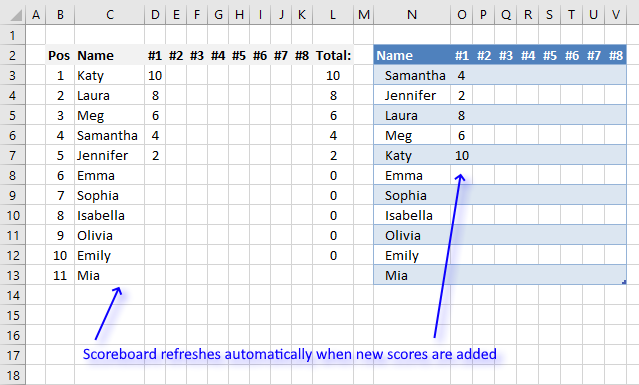This article demonstrates a scoreboard, displayed to the left, that sorts contestants based on total scores and refreshes instantly each time you enter or edit scores.

This entire scoreboard is based on formulas only and it is the amazing MMULT function that makes it possible.

The Excel defined Table, shown to the right in the picture above, allows you to enter as many names as you want, no need to update cell references in formulas.

## 1. Dynamic scoreboardArray formula in cell C1:

=IFERROR(INDEX(Table2, MATCH(LARGE(MMULT(IF(Table2[['#1]:['#8]]<>"", Table2[['#1]:['#8]], 0), TRANSPOSE(COLUMN(Table2[[#Headers], ['#1]:['#8]])^0)), ROWS(\$A\$1:A1)), IF((COUNTIF(\$C\$2:C2, Table2[Name])=0), MMULT(IF(Table2[['#1]:['#8]]<>"", Table2[['#1]:['#8]], 0), TRANSPOSE(COLUMN(Table2[[#Headers], ['#1]:['#8]])^0)), "A"), 0), COLUMNS(\$A\$1:A1)), "")

The formula works even if two or more people share the same total score.

### How to enter an array formula

An array formula is a regular formula on steroids, it allows you to perform much more complicated calculations with amazing results.

To enter an array formula you must follow these steps:

1. Copy array formula above (CTRL + c).
2. Double press with left mouse button on cell C1.
3. Paste array formula to the cell.
4. Press and hold CTRL + SHIFT simultaneously.
5. Press Enter once.
6. Release all keys.

If you did it right the formula in the formula bar now begins and ends with curly brackets, like this ={array_formula}. Don't type these characters yourself, they appear if the above steps were correctly done.

Note, if a user edits an array formula and then press Enter key on the keyboard the array formula becomes a regular formula, however, if a user press Esc key on the keyboard the formula returns to an array formula.

This is the single most annoying thing with array formulas, users can easily break them.

### How to create an Excel Table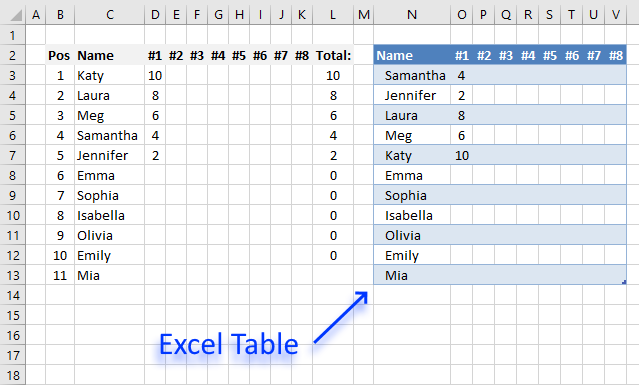The Excel Table has many useful features, in this case, one specific feature is really great. It allows you to add or delete names to the scoreboard without adjusting cell references, you can have as many names as you like in the Excel Table.

1. Select any cell in your data set.
2. Press shortcut keys CTRL + T to display the Excel Table dialog box.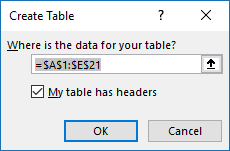3. Press with left mouse button on OK button to apply settings and create the table.

### Hiding zeros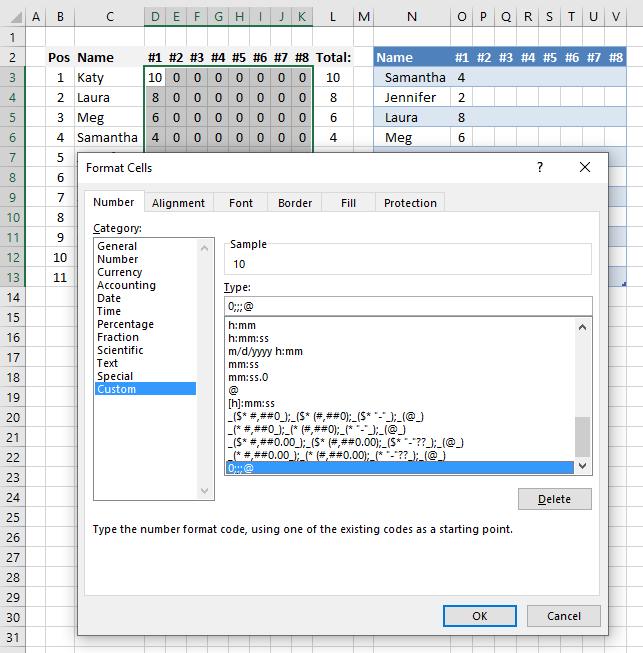I have applied custom cell formatting to cell range D3:K7 in order to hide 0's (zeros), you can skip these steps if you want them in your worksheet.

1. Select cell range D3:K7
2. Press short cut keys CTRL + 1 to open the "Format Cells" dialog box.
3. Go to tab "Number".
4. Press with left mouse button on "Custom" category.
5. Type: 0;-0;;@
6. Press with left mouse button on OK button to apply cell formatting.

### Explaining formula in cell C3

#### Step 1 - Check if scores are empty

This step creates an array based on cell range D3:K7 and replaces blank values with 0's (zeros).

The less than and greater than characters combined means not equal to and in this case we compare nothing "" meaning an empty cell.

Table2[['#1]:['#8]] is a structured reference pointing to columns #1 to #8 in Table2, Table2 is the name of the Excel Table.

Table2[['#1]:['#8]]<>""

becomes

{10, "", "", "", "", "", "", "";8, "", "", "", "", "", "", "";6, "", "", "", "", "", "", "";4, "", "", "", "", "", "", "";2, "", "", "", "", "", "", "";"", "", "", "", "", "", "", "";"", "", "", "", "", "", "", "";"", "", "", "", "", "", "", "";"", "", "", "", "", "", "", "";"", "", "", "", "", "", "", "";"", "", "", "", "", "", "", ""}<>""

and returns

{TRUE, FALSE, FALSE, FALSE, FALSE, FALSE, FALSE, FALSE;TRUE, FALSE, FALSE, FALSE, FALSE, FALSE, FALSE, FALSE;TRUE, FALSE, FALSE, FALSE, FALSE, FALSE, FALSE, FALSE;TRUE, FALSE, FALSE, FALSE, FALSE, FALSE, FALSE, FALSE;TRUE, FALSE, FALSE, FALSE, FALSE, FALSE, FALSE, FALSE;FALSE, FALSE, FALSE, FALSE, FALSE, FALSE, FALSE, FALSE;FALSE, FALSE, FALSE, FALSE, FALSE, FALSE, FALSE, FALSE;FALSE, FALSE, FALSE, FALSE, FALSE, FALSE, FALSE, FALSE;FALSE, FALSE, FALSE, FALSE, FALSE, FALSE, FALSE, FALSE;FALSE, FALSE, FALSE, FALSE, FALSE, FALSE, FALSE, FALSE;FALSE, FALSE, FALSE, FALSE, FALSE, FALSE, FALSE, FALSE}

The IF function can now, based on the above array, return a specific value if TRUE and another value if FALSE.

IF(Table2[['#1]:['#8]]<>"", Table2[['#1]:['#8]], 0)

becomes

IF({TRUE, FALSE, FALSE, FALSE, FALSE, FALSE, FALSE, FALSE;TRUE, FALSE, FALSE, FALSE, FALSE, FALSE, FALSE, FALSE;TRUE, FALSE, FALSE, FALSE, FALSE, FALSE, FALSE, FALSE;TRUE, FALSE, FALSE, FALSE, FALSE, FALSE, FALSE, FALSE;TRUE, FALSE, FALSE, FALSE, FALSE, FALSE, FALSE, FALSE;FALSE, FALSE, FALSE, FALSE, FALSE, FALSE, FALSE, FALSE;FALSE, FALSE, FALSE, FALSE, FALSE, FALSE, FALSE, FALSE;FALSE, FALSE, FALSE, FALSE, FALSE, FALSE, FALSE, FALSE;FALSE, FALSE, FALSE, FALSE, FALSE, FALSE, FALSE, FALSE;FALSE, FALSE, FALSE, FALSE, FALSE, FALSE, FALSE, FALSE;FALSE, FALSE, FALSE, FALSE, FALSE, FALSE, FALSE, FALSE}, {10, "", "", "", "", "", "", "";8, "", "", "", "", "", "", "";6, "", "", "", "", "", "", "";4, "", "", "", "", "", "", "";2, "", "", "", "", "", "", "";"", "", "", "", "", "", "", "";"", "", "", "", "", "", "", "";"", "", "", "", "", "", "", "";"", "", "", "", "", "", "", "";"", "", "", "", "", "", "", "";"", "", "", "", "", "", "", ""}, 0)

and returns

{4, 0, 0, 0, 0, 0, 0, 0;2, 0, 0, 0, 0, 0, 0, 0;8, 0, 0, 0, 0, 0, 0, 0;6, 0, 0, 0, 0, 0, 0, 0;10, 0, 0, 0, 0, 0, 0, 0;0, 0, 0, 0, 0, 0, 0, 0;0, 0, 0, 0, 0, 0, 0, 0;0, 0, 0, 0, 0, 0, 0, 0;0, 0, 0, 0, 0, 0, 0, 0;0, 0, 0, 0, 0, 0, 0, 0;0, 0, 0, 0, 0, 0, 0, 0}

#### Step 2 - Create column numbers based on Excel Table headers

The COLUMN function returns a column number based on a cell reference.

becomes

COLUMN(O2:V2)

and returns {15, 16, 17, 18, 19, 20, 21, 22} which means that the headers are located in column number 15 to 22.

#### Step 3 - Transpose numbers

The array size is correct, however, all numbers must be 1 to be able to use them in the MMULT function in order to return the desired result. To achieve that we can calculate powers of numbers, in this case power of 0 (zero) which will return 1.

becomes

TRANSPOSE({15, 16, 17, 18, 19, 20, 21, 22}^0)

becomes

TRANSPOSE({1, 1, 1, 1, 1, 1, 1, 1})

To be able to use the numbers with the MMULT function they need to be separated by semicolons which is easy to accomplish, the TRANSPOSE function changes the array from being arranged horizontally to vertically.

TRANSPOSE({1, 1, 1, 1, 1, 1, 1, 1})

returns

{1; 1; 1; 1; 1; 1; 1; 1}

#### Step 4 - Add numbers column-wise

The MMULT function allows us to sum the numbers horizontally using two arrays.

MMULT(IF(Table2[['#1]:['#8]]<>"", Table2[['#1]:['#8]], 0), TRANSPOSE(COLUMN(Table2[[#Headers], ['#1]:['#8]])^0))

becomes

MMULT({4, 0, 0, 0, 0, 0, 0, 0;2, 0, 0, 0, 0, 0, 0, 0;8, 0, 0, 0, 0, 0, 0, 0;6, 0, 0, 0, 0, 0, 0, 0;10, 0, 0, 0, 0, 0, 0, 0;0, 0, 0, 0, 0, 0, 0, 0;0, 0, 0, 0, 0, 0, 0, 0;0, 0, 0, 0, 0, 0, 0, 0;0, 0, 0, 0, 0, 0, 0, 0;0, 0, 0, 0, 0, 0, 0, 0;0, 0, 0, 0, 0, 0, 0, 0}, TRANSPOSE(COLUMN(Table2[[#Headers], ['#1]:['#8]])^0))

becomes

MMULT({4, 0, 0, 0, 0, 0, 0, 0;2, 0, 0, 0, 0, 0, 0, 0;8, 0, 0, 0, 0, 0, 0, 0;6, 0, 0, 0, 0, 0, 0, 0;10, 0, 0, 0, 0, 0, 0, 0;0, 0, 0, 0, 0, 0, 0, 0;0, 0, 0, 0, 0, 0, 0, 0;0, 0, 0, 0, 0, 0, 0, 0;0, 0, 0, 0, 0, 0, 0, 0;0, 0, 0, 0, 0, 0, 0, 0;0, 0, 0, 0, 0, 0, 0, 0}, {1; 1; 1; 1; 1; 1; 1; 1})

and returns {4; 2; 8; 6; 10; 0; 0; 0; 0; 0; 0}. The numbers in the array are in the same order as the names in the Excel Table which is neccessary to be able to sort the names based on scores.

#### Step 5 - Extract the k-th largest score

The LARGE function calculates the k-th largest number in the array. LARGE(array, k)

LARGE(MMULT(IF(Table2[['#1]:['#8]]<>"", Table2[['#1]:['#8]], 0), TRANSPOSE(COLUMN(Table2[[#Headers], ['#1]:['#8]])^0)), ROWS(\$A\$1:A1))

becomes

LARGE({4; 2; 8; 6; 10; 0; 0; 0; 0; 0; 0}, ROWS(\$A\$1:A1))

The ROWS function contains a cell reference that is both absolute and relative, this makes the cell reference grow when the formula is copied to cells below. This is needed in order to extract a new value in each cell.

LARGE({4; 2; 8; 6; 10; 0; 0; 0; 0; 0; 0}, ROWS(\$A\$1:A1))

becomes

LARGE({4; 2; 8; 6; 10; 0; 0; 0; 0; 0; 0}, 1)

and returns 10.

#### Step 6 - Find relative position in array

We have now calculated the k-th largest number in the array and this step explains how to find the position of that value in the array. The MATCH function allows us to do that.

MATCH(LARGE(MMULT(IF(Table2[['#1]:['#8]]<>"", Table2[['#1]:['#8]], 0), TRANSPOSE(COLUMN(Table2[[#Headers], ['#1]:['#8]])^0)), ROWS(\$A\$1:A1)), IF((COUNTIF(\$C\$2:C2, Table2[Name])=0), MMULT(IF(Table2[['#1]:['#8]]<>"", Table2[['#1]:['#8]], 0), TRANSPOSE(COLUMN(Table2[[#Headers], ['#1]:['#8]])^0)), "A"), 0)

becomes

MATCH(10, IF((COUNTIF(\$C\$2:C2, Table2[Name])=0), MMULT(IF(Table2[['#1]:['#8]]<>"", Table2[['#1]:['#8]], 0), TRANSPOSE(COLUMN(Table2[[#Headers], ['#1]:['#8]])^0)), "A"), 0)

becomes

MATCH(10, {4; 2; 8; 6; 10; 0; 0; 0; 0; 0; 0}, 0)

and returns 5. Number 10 is found in position 5 in the array.

#### Step 7 - Extract the corresponding record to the calculated position

The INDEX function lets you fetch a value based on row and column number.

INDEX(Table2, MATCH(LARGE(MMULT(IF(Table2[['#1]:['#8]]<>"", Table2[['#1]:['#8]], 0), TRANSPOSE(COLUMN(Table2[[#Headers], ['#1]:['#8]])^0)), ROWS(\$A\$1:A1)), IF((COUNTIF(\$C\$2:C2, Table2[Name])=0), MMULT(IF(Table2[['#1]:['#8]]<>"", Table2[['#1]:['#8]], 0), TRANSPOSE(COLUMN(Table2[[#Headers], ['#1]:['#8]])^0)), "A"), 0), COLUMNS(\$A\$1:A1))

becomes

INDEX(Table2, 10, COLUMNS(\$A\$1:A1))

The COLUMNS function chnages when the formula is copied to cells located to the right, this lets us extract the entire record.

INDEX(Table2, 10, COLUMNS(\$A\$1:A1))

becomes

INDEX(Table2, 10, 1)

and returns "Katy" in cell C3.

#### Step 8 - Remove errors

The IFERROR function removes errors that will show up when all names have been displayed.

IFERROR(INDEX(Table2, MATCH(LARGE(MMULT(IF(Table2[['#1]:['#8]]<>"", Table2[['#1]:['#8]], 0), TRANSPOSE(COLUMN(Table2[[#Headers], ['#1]:['#8]])^0)), ROWS(\$A\$1:A1)), IF((COUNTIF(\$C\$2:C2, Table2[Name])=0), MMULT(IF(Table2[['#1]:['#8]]<>"", Table2[['#1]:['#8]], 0), TRANSPOSE(COLUMN(Table2[[#Headers], ['#1]:['#8]])^0)), "A"), 0), COLUMNS(\$A\$1:A1)), "")

Note, the IFERROR function removes all kinds of errors which may make it difficult to spot other errors in the formula. Use with caution.

Interested in learning more about array formulas? Join my Advanced Excel Course.

### Animated image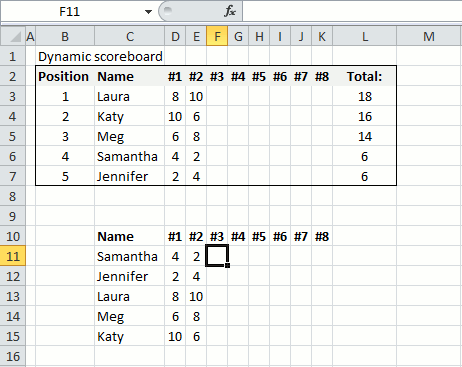The image above shows the scoreboard while I am updating the scores, it sorts the names based on the total.

### Get the Excel fileDynamic-scoreboard-Excel-Table.xlsx

## 2. Dynamic scoreboard - Excel 365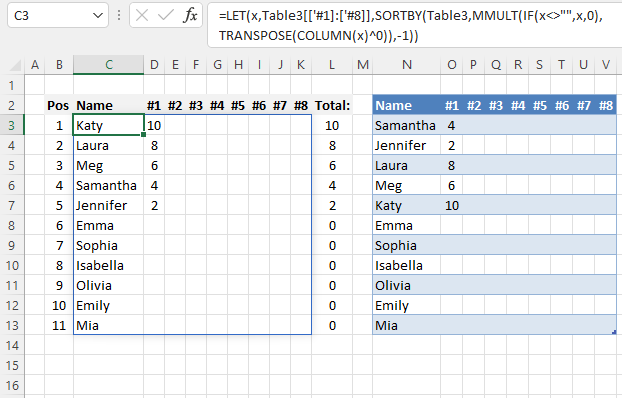Excel 365 lets you use new functions that are better and the formula is a lot shorter. Type the formula in cell C3 and simply press enter. The formula spills to cells below and to the right as far as needed.

Dynamic array formula in cell C3:

=LET(x,Table3[['#1]:['#8]],SORTBY(Table3,MMULT(IF(x<>"",x,0),TRANSPOSE(COLUMN(x)^0)),-1))

### Explaining formula

#### Step 1 - Check if not empty

Table3[['#1]:['#8]]<>""

#### Step 2 - Replace empty values with 0 (zero)

IF(Table3[['#1]:['#8]]<>"",Table3[['#1]:['#8]],0)

#### Step 3 - Create column numbers based on the structured reference

COLUMN(Table3[['#1]:['#8]])

#### Step 4 - Convert numbers to 1

COLUMN(Table3[['#1]:['#8]])^0

#### Step 5 - Rearrange horizontal values to vertical

TRANSPOSE(COLUMN(Table3[['#1]:['#8]])^0))

#### Step 6 - Sum numbers row-wise

MMULT(IF(Table3[['#1]:['#8]]<>"",Table3[['#1]:['#8]],0),TRANSPOSE(COLUMN(Table3[['#1]:['#8]])^0))

#### Step 7 - Sort the table based on the sum

SORTBY(Table3,MMULT(IF(Table3[['#1]:['#8]]<>"",Table3[['#1]:['#8]],0),TRANSPOSE(COLUMN(Table3[['#1]:['#8]])^0)),-1)

#### Step 8 - Shorten the formula

SORTBY(Table3,MMULT(IF(Table3[['#1]:['#8]]<>"",Table3[['#1]:['#8]],0),TRANSPOSE(COLUMN(Table3[['#1]:['#8]])^0)),-1)

x - Table3[['#1]:['#8]]

LET(x,Table3[['#1]:['#8]],SORTBY(Table3,MMULT(IF(x<>"",x,0),TRANSPOSE(COLUMN(x)^0)),-1))